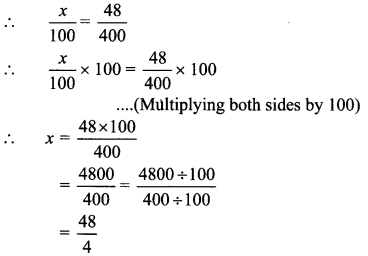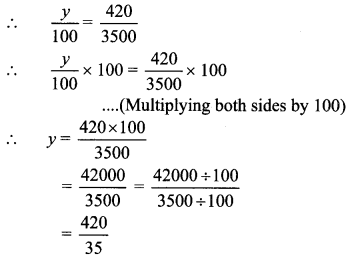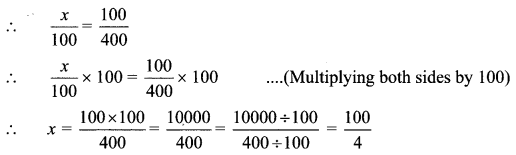# Maharashtra Board Practice Set 33 Class 6 Maths Solutions Chapter 13 Profit-Loss

Balbharti Maharashtra State Board Class 6 Maths Solutions covers the Std 6 Maths Chapter 13 Profit-Loss Class 6 Practice Set 33 Answers Solutions.

## 6th Standard Maths Practice Set 33 Answers Chapter 13 Profit-Loss

Question 1.
Maganlal bought trousers for Rs 400 and a shirt for Rs 200 and sold them for Rs 448 and Rs 250 respectively. Which of these transactions was more profitable?
Solution:
Cost price of trousers = Rs 400
Selling price of trousers = Rs 448
Profit = Selling price – Cost price
= 448 – 400 = Rs 48
Let Maganlal make x % profit on selling trousers∴ x = 12%
Cost price of shirt = Rs 200
Selling price of shirt = Rs 250
∴ Profit = Selling price – Cost price
= 250 – 200
= Rs 50
Let Maganlal make y% profit on selling shirt.∴ y = 25%
∴ Transaction involving selling of shirt was more profitable.

Question 2.
Ramrao bought a cupboard for Rs 4500 and sold it for Rs 4950. Shamrao bought a sewing machine for Rs 3500 and sold it for Rs 3920. Whose transaction was more profitable?
Solution:
Cost price of cupboard = Rs 4500
Selling price of cupboard = Rs 4950
∴ Profit = Selling price – Cost price
= 4950 – 4500
= Rs 450
Let Ramrao make x% profit on selling cupboard∴ x = 10%
Cost price of sewing machine = Rs 3500
Selling price of sewing machine = Rs 3920
∴Profit = Selling price – Cost price
= 3920 – 3500
= Rs 420
Shamrao make y% profit on selling sewing machine.∴y = 12%
∴Shamrao’s transaction was more profitable.

Question 3.
Hanif bought one box of 50 apples for Rs 400. He sold all the apples at the rate of Rs 10 each. Was there a profit or loss? What was its percentage?
Solution:
Cost price of 50 apples = Rs 400
Selling price of one apple = Rs 10
∴ Selling price of 50 apples = 10 x 50 = Rs 500
Selling price is greater than the total cost price.